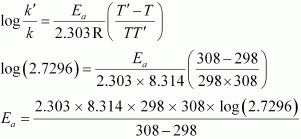Q

# The time required for 10% completion of a first order reaction at 298K is equal to that required for its 25% completion at 308K. If the value of A is 4 × 10 ^ 10 s ^ –1. Calculate k at 318K and Ea.

4.29   The time required for 10% completion of a first order reaction at 298K is equal to that required for its 25% completion at 308K. If the value of A is . Calculate k at 318K and Ea.

Views

We know that,

for a first order reaction-

Case 1
At temp. = 298 K

= 0.1054/k

Case 2
At temp = 308 K

= 2.2877/k'
As per the question

K'/K = 2.7296

From Arrhenius equation,= 76640.096 J /mol
=76.64 KJ/mol

k at 318 K
we have , T =318K
A=

Now
After putting the calue of given variable, we get

on takingantilog we get,

k = antilog(-1.9855)

= 1.034

Exams
Articles
Questions